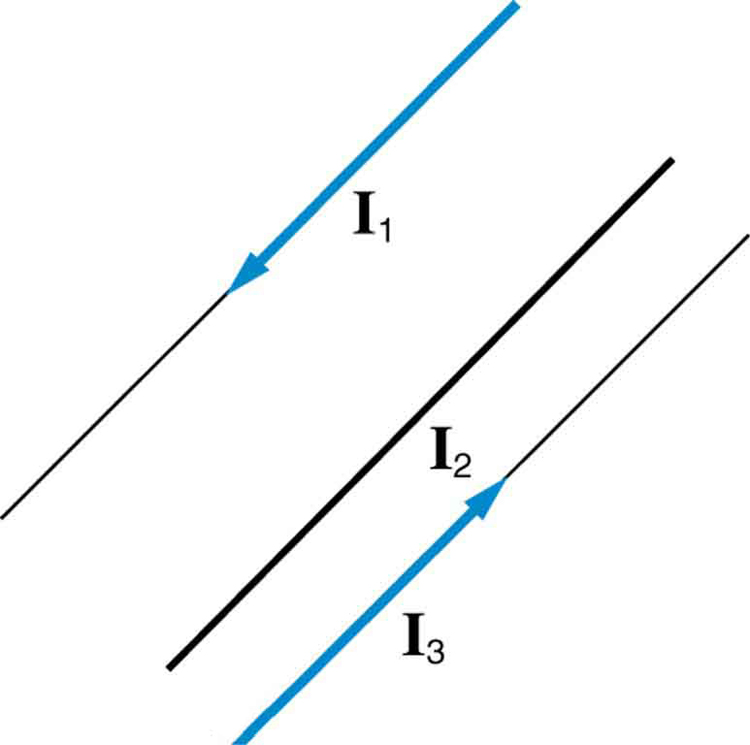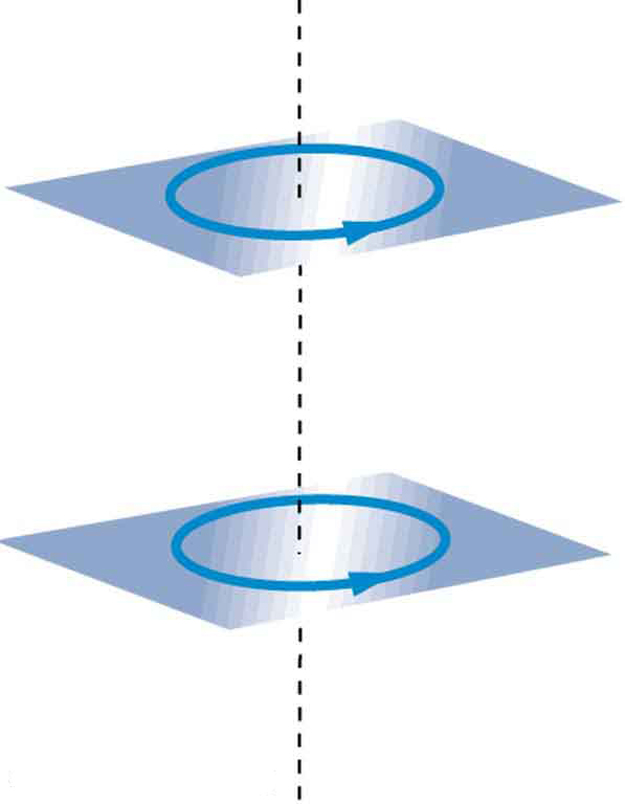# 22.10 Magnetic force between two parallel conductors  (Page 2/2)

 Page 2 / 2

Since ${\mu }_{0}$ is exactly $4\pi ×{\text{10}}^{-7}\phantom{\rule{0.25em}{0ex}}T\cdot \text{m/A}$ by definition, and because $\text{1 T}=\text{1 N/}\left(A\cdot m\right)$ , the force per meter is exactly $2×{\text{10}}^{-7}\phantom{\rule{0.25em}{0ex}}\text{N/m}$ . This is the basis of the operational definition of the ampere.

## The ampere

The official definition of the ampere is:

One ampere of current through each of two parallel conductors of infinite length, separated by one meter in empty space free of other magnetic fields, causes a force of exactly $2×{\text{10}}^{-7}\phantom{\rule{0.25em}{0ex}}\text{N/m}$ on each conductor.

Infinite-length straight wires are impractical and so, in practice, a current balance is constructed with coils of wire separated by a few centimeters. Force is measured to determine current. This also provides us with a method for measuring the coulomb. We measure the charge that flows for a current of one ampere in one second. That is, $1 C=1 A\cdot s$ . For both the ampere and the coulomb, the method of measuring force between conductors is the most accurate in practice.

## Section summary

• The force between two parallel currents ${I}_{1}$ and ${I}_{2}$ , separated by a distance $r$ , has a magnitude per unit length given by
$\frac{F}{l}=\frac{{\mu }_{0}{I}_{1}{I}_{2}}{2\mathrm{\pi r}}.$
• The force is attractive if the currents are in the same direction, repulsive if they are in opposite directions.

## Conceptual questions

Is the force attractive or repulsive between the hot and neutral lines hung from power poles? Why?

If you have three parallel wires in the same plane, as in [link] , with currents in the outer two running in opposite directions, is it possible for the middle wire to be repelled by both? Attracted by both? Explain.Three parallel coplanar wires with currents in the outer two in opposite directions.

Suppose two long straight wires run perpendicular to one another without touching. Does one exert a net force on the other? If so, what is its direction? Does one exert a net torque on the other? If so, what is its direction? Justify your responses by using the right hand rules.

Use the right hand rules to show that the force between the two loops in [link] is attractive if the currents are in the same direction and repulsive if they are in opposite directions. Is this consistent with like poles of the loops repelling and unlike poles of the loops attracting? Draw sketches to justify your answers.Two loops of wire carrying currents can exert forces and torques on one another.

If one of the loops in [link] is tilted slightly relative to the other and their currents are in the same direction, what are the directions of the torques they exert on each other? Does this imply that the poles of the bar magnet-like fields they create will line up with each other if the loops are allowed to rotate?

Electric field lines can be shielded by the Faraday cage effect. Can we have magnetic shielding? Can we have gravitational shielding?

## Problems&Exercises

(a) The hot and neutral wires supplying DC power to a light-rail commuter train carry 800 A and are separated by 75.0 cm. What is the magnitude and direction of the force between 50.0 m of these wires? (b) Discuss the practical consequences of this force, if any.

(a) 8.53 N, repulsive

(b) This forceis repulsiveand thereforethere isnever arisk thatthe twowires willtouch andshort circuit.

The force per meter between the two wires of a jumper cable being used to start a stalled car is 0.225 N/m. (a) What is the current in the wires, given they are separated by 2.00 cm? (b) Is the force attractive or repulsive?

A 2.50-m segment of wire supplying current to the motor of a submerged submarine carries 1000 A and feels a 4.00-N repulsive force from a parallel wire 5.00 cm away. What is the direction and magnitude of the current in the other wire?

400 A in the opposite direction

The wire carrying 400 A to the motor of a commuter train feels an attractive force of $4\text{.}\text{00}×{\text{10}}^{-3}\phantom{\rule{0.25em}{0ex}}\text{N/m}$ due to a parallel wire carrying 5.00 A to a headlight. (a) How far apart are the wires? (b) Are the currents in the same direction?

An AC appliance cord has its hot and neutral wires separated by 3.00 mm and carries a 5.00-A current. (a) What is the average force per meter between the wires in the cord? (b) What is the maximum force per meter between the wires? (c) Are the forces attractive or repulsive? (d) Do appliance cords need any special design features to compensate for these forces?

(a) $1\text{.}\text{67}×{\text{10}}^{-3}\phantom{\rule{0.25em}{0ex}}\text{N/m}$

(b) $3\text{.}\text{33}×{\text{10}}^{-3}\phantom{\rule{0.25em}{0ex}}\text{N/m}$

(c) Repulsive

(d) No, these are very small forces

[link] shows a long straight wire near a rectangular current loop. What is the direction and magnitude of the total force on the loop?

Find the direction and magnitude of the force that each wire experiences in [link] (a) by, using vector addition.

(a) Top wire: $2\text{.}\text{65}×{\text{10}}^{-4}\phantom{\rule{0.25em}{0ex}}\text{N/m}$ s, $\text{10}\text{.}9º$ to left of up

(b) Lower left wire: $3\text{.}\text{61}×{\text{10}}^{-4}\phantom{\rule{0.25em}{0ex}}\text{N/m}$ , $\text{13}\text{.}9º$ down from right

(c) Lower right wire: $3\text{.}\text{46}×{\text{10}}^{-4}\phantom{\rule{0.25em}{0ex}}\text{N/m}$ , $\text{30}\text{.}0º$ down from left

Find the direction and magnitude of the force that each wire experiences in [link] (b), using vector addition.

full meaning of GPS system
how to prove that Newton's law of universal gravitation F = GmM ______ R²
sir dose it apply to the human system
prove that the centrimental force Fc= M1V² _________ r
prove that centripetal force Fc = MV² ______ r
Kaka
how lesers can transmit information
griffts bridge derivative
below me
please explain; when a glass rod is rubbed with silk, it becomes positive and the silk becomes negative- yet both attracts dust. does dust have third types of charge that is attracted to both positive and negative
what is a conductor
Timothy
hello
Timothy
below me
why below you
Timothy
no....I said below me ...... nothing below .....ok?
dust particles contains both positive and negative charge particles
Mbutene
corona charge can verify
Stephen
when pressure increases the temperature remain what?
remains the temperature
betuel
what is frequency
define precision briefly
CT scanners do not detect details smaller than about 0.5 mm. Is this limitation due to the wavelength of x rays? Explain.
hope this helps
what's critical angle
The Critical Angle Derivation So the critical angle is defined as the angle of incidence that provides an angle of refraction of 90-degrees. Make particular note that the critical angle is an angle of incidence value. For the water-air boundary, the critical angle is 48.6-degrees.
okay whatever
Chidalu
pls who can give the definition of relative density?
Temiloluwa
the ratio of the density of a substance to the density of a standard, usually water for a liquid or solid, and air for a gas.
Chidalu
What is momentum
mass ×velocity
Chidalu
it is the product of mass ×velocity of an object
Chidalu
how do I highlight a sentence]p? I select the sentence but get options like copy or web search but no highlight. tks. src
then you can edit your work anyway you want
Wat is the relationship between Instataneous velocity
Instantaneous velocity is defined as the rate of change of position for a time interval which is almost equal to zero
AstronomyByBy OpenStaxBy OpenStaxBy OpenStaxBy Stephen VoronBy Maureen MillerBy OpenStaxBy OpenStaxBy OpenStaxBy Stephanie RedfernBy George Turner